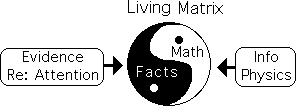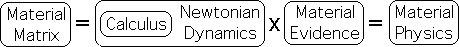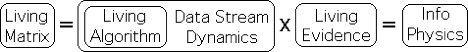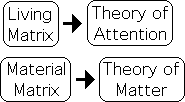# Math-Fact Matrix

### Living Matrix -> Theory of Attention

#### Math/Fact Matrix -> Info Physics

My Theory of Attention is based upon a Math/Fact Matrix – the Living Matrix, to be precise.

To facilitate a common understanding, let us provide some definitions.

A Math/Fact Matrix is a complex web of interlocking relationships between a mathematical system and scientific facts.The Material Matrix is the most famous, as it is at the heart of our technological wonderland. Newtonian Physics provides the mathematics while material behavior provides the evidence.The Living Matrix is another type of Math/Fact Matrix. The Physics of Information provides the mathematical system, while evidence surrounding Attention provide the scientific facts. Another intent of this volume is to establish the validity of the Living Matrix.### Scientific Facts: Universally accepted Phenomena, Models or Theories

#### Definitions: Components of MF Matrix’s

Let’s define the components of our Math/Fact Matrix.

#### Definition: Scientific Fact: Universally accepted as true

Fact, in this limited context, only refers to scientific facts. We use the term broadly to indicate any kind of belief that is widely held by scientists in any field. There must be virtually universal acceptance of the belief’s validity amongst the scientific community. The certainty must be so strong that the belief is generally taken as a fact. In other words, scientific facts are accepted as unquestionably true.

#### Scientific Facts Types: Simple, Finding, Complex Theory

Scientific facts can be take many forms: 1) a simple measurement, e.g. that board is 2” by 4”, 2) simple experimental findings, e.g. a water molecule consists of 2 hydrogen atoms and 1 oxygen atom; 3) complex living behaviors, e.g. sleep deprivation harms cognitive performance, or 4) a complex theory, e.g. Newton’s law of gravity and Darwin’s theory of evolution. A general scientific fact that is pertinent to our study: evolutionary forces determine biological forms.

#### SF identifies and defines Phenomenon or Interactions

These facts generally define a phenomenon or its interactions with other phenomenon. The phenomenon can be specific or general. Humans have two eyes; living systems are composed of cells; cells cooperate as a group to create organisms.

Reiterating for retention, the Physics of Attention derived from a Math/Fact Matrix. The facts in this matrix are not just numerical measurements, but instead beliefs that are universally held by the scientific community – Scientific Facts.

### Matrix: Mathematical Systems

What about the Math part of the matrix?

#### Definition: Math = Math system that coincides with Data

The mathematics of a Math/Fact Matrix is a system. In order to be considered part of a matrix, this system must coincide with a set of scientific facts. This type of mathematical system can take many forms.

#### Material Matrix: Calculus, Newtonian Dynamics x Material Evidence = Physics of Matter

The most spectacular is Newtonian Dynamics, which provides the dynamical structure of the so-called Material Matrix. Calculus provides the mathematical structure of the system. The interaction between the math system and material evidence gave rise to the Material Matrix, i.e. the Physics of Matter.#### Living Matrix: DSD, Living Algorithm x Living Evidence = Info Physics

Data Stream Dynamics (DSD) provides the physics, i.e. the dynamical structure, for our particular matrix – the so-called Living Matrix. The Living Algorithm (LA) provides the mathematical structure for DSD. The interaction between the math system and living evidence gave rise to the Living Matrix, i.e. the Physics of Information.#### Closed & Regular Equations vs. Open & Reflexive Equations

The Physics of Matter and the Physics of Information are entirely different in that they belong to mutually exclusive sets. Calculus is based in Closed and Regular Equations. In other words, these equations are closed to external input and don’t refer to themselves. In contrast, the Living Algorithm is an Open and Reflexive Equation. Put another way, the LA is open to external input and past results play a part in determining current results.

#### Living Matrix > Theory of Attention

The Living Matrix gave birth to my Theory of Attention. The Material Matrix gave birth to the Theory of Matter. The Theory of Matter is so well-established that it could be considered a scientific fact. On the other hand, my Theory of Attention has a fan base of one. It is for this reason that I have written so many supportive pages.### Math correspondences with Scientific Facts: Types of Linkages

A Math/Fact Matrix indicates that there are distinct patterns of correspondence between a mathematical system and scientific facts. How is the correspondence validated or verified?

According to my Theory of Attention, there are at least 3 mathematical realms of existence. Each realm has a different relationship with the facts. Let us explore the nature of these relationships one realm at a time.

#### Molecular Realm: direct observable phenomena & numerical data

1) Molecular Realm: Scientists access this realm through direct observation. They first accumulate precise measurements regarding the absolute essences of particles/objects as they move through space. These measurements are rendered in numbers. For instance, an iron ball weighs 2 pounds and is 4 inches in diameter. Equations are then written to match the numerical patterns. Validated formulas become scientific facts, e.g. E = mc2. In this realm, objects, even atoms, can be observed moving through space and time.

#### Subatomic Realm: indirect observations & numerical data

2) Subatomic Realm: In contrast, scientists can only infer the existence of this realm through indirect observation. Because Subatomic entities, such as electrons and photons, are not observable, scientists deduce their existence by their effects. For instance, sound or visual trails are examined to ascertain the characteristics of the bizarre inhabitants of this peculiar realm. This data is also numerical.

#### Realm of Attention: visual pattern recognition, no numerical data

3) Living Realm of Attention: Like the other realms of existence, the Math/Fact matrix is based upon patterns of correspondence between phenomena that are established scientific facts and a mathematical system. However, the inferences regarding the characteristics of this realm are generally based upon visually based evidence. Rather than numerical data, we employ visual pattern recognition in order to establish the correspondences. Put another way, an examination of the mathematical behavior of LA’s model reveals similarities with living behavior.

### 3 Factors validate Attention Matrix

#### Altho’ lacking rigor, Attention Matrix validated other ways

It is evident that the Math-Fact Matrix associated with Attention lacks the empirical rigor of the Material Matrices. Despite this deficiency, three factors establish the validity of the Attention Matrix: 1) It provides explanatory power for a vast range of phenomena that remain scientific mysteries. 2) The Math and Fact network are isometric systems in that they share a common underlying inferential structure, i.e. exhibit a high degree of logical symmetry, and 3) redundancy logic, i.e. cross-validation from multiple sources. These same factors validate each Math-Fact Matrix.#### Explanatory Power for Scientific Mysteries

Let us provide a brief introduction to each factor in the so-called validation network for the Attention Matrix starting with explanatory power. Our model provides explanatory power for a wide range of phenomena from a variety of disciplines. Some of these experimentally verified phenomena remain scientific mysteries despite intensive research and speculation. The community understands ‘What’ happens, but have yet to uncover the ‘Why’ behind these enigmatic phenomena. Conversely, our mathematical model provides plausible explanations that link countless phenomena from a multiple disciplines under one roof.

#### Isomorphic Logic

What does the roof consist of? And what binds it together? Isometric logic: the process of employing the implicit logical structure of a known system to better understand the implicit logical structure of an unknown system. Humans use this process to form verbal abstractions; scientists use this process as a powerful tool. They employ the implicit logic of mathematical systems to better understand of implicit logic of empirical systems. As another form of validation, the LA system has an isomorphic relationship with a network of phenomena associated with Attention.

#### Redundancy Logic: cross-disciplinary validation

Redundancy Logic is the final factor validating the Math-Fact Matrix that defines the Realm of Attention. According to this extraordinary type of logic: the greater the number of sources pointing to the same conclusion, the more likely the conclusion is true. Living systems employ this form of logic to affirm a common reality. Scientists employ Redundancy Logic as a means of forming the common consensus that is the foundation of validating scientific theory.

#### Redundancy Logic supports Realm of Attention

1) There are an uncountable number of patterns of correspondences between scientific facts and the LA’s mathematical system. 2) These scientific facts span a wide range of phenomena from a variety of disciplines. 3) DSD provides explanatory power for many scientific mysteries. 4) DSD and a phenomenal system have an isometric relationship, i.e. a high degree of logical symmetry. Each of these sources support the conclusion that DSD provides a good model for the network of phenomena associated with the Realm of Attention. The redundancy of these multiple forms of evidence provides overwhelming support for our model.

#### Matches common sense: 3 Logical Redundancies > Indestructible web of understanding

Finally, our Theory of Attention matches and extends our common sense notions regarding intention, mental energy, and choice. With this addition, there are three forms of validation for the conclusions behind the Theory of Attention: 1) cross-disciplinary evidence linked under one roof, 2) explanatory power for scientific mysteries, and 3) congruence with our common sense regarding choice. This three part logical redundancy generates a virtually indestructible logical web of understanding.#### Significance:  maximize possibility of fulfilling potentials

What is the significance of my work?

Attention Theory provides a theoretical foundation for our common sense understanding of choice. The mathematically based theory integrates intention, information, attention, mental energy and experience in an elegant package that embodies simplicity. Its significance lies in its ability to extend our understanding of Attention. With deeper insights into this fundamental feature of living experience, we can maximize the quality of our lives. With this knowledge, we can more easily both fulfill potentials and avoid pitfalls.

#### Book’s intent: validating Math/Fact Matrix that is theory’s foundation

As mentioned, the Living Matrix provides the foundation for my Theory of Attention. Due to its foundational importance, this entire work is devoted to establishing the validity of the matrix. To that end, we examine the myriad patterns of correspondence between living behavior and the mathematical behavior of our model. More specifically, we examine the parallels between well-established Attention and Sleep related phenomena and the behavior of the two primary forms of our mathematical system, the Pulse and the Triple Pulse. As will be exhibited, there are widespread patterns of correspondence between the mathematical behavior of these two forms and many aspects of living behavior, especially those regarding Attention.

#### Provocative Questions

You are probably wondering, how could mathematics possibly apply to imprecise, unpredictable living behavior? If it does apply to behavior, what are the implications? Could an understanding of the dynamics of behavior actually improve mental performance and the overall quality of life? Could the employment of these mathematical mechanisms in our day-to-day life actually improve our chances of having an 'optimal experience' - moving into the ‘Zone’ – becoming ‘one with the Tao’?